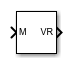# MATLAB to VR Coordinates

(To be removed) Convert MATLAB coordinates to VR coordinates

Since R2019a

MATLAB to VR Coordinates will be removed in a future release. For more information, see Version History.

•Libraries:

## Description

The MATLAB to VR Coordinates block converts a point with coordinates in the MATLAB® coordinate system to the VRML coordinate system.

The following relation holds between the two coordinate systems:

[xm, ym, zm] = [xv, zv, -yv]

where MATLAB coordinates are denoted with the m subscript and Virtual World coordinates are denoted with the v subscript. For more information on the two coordinate systems, see Virtual World Coordinate System.

## Ports

### Input

expand all

Coordinates of a point in MATLAB notation, specified as a 3-element row vector.

Data Types: `single` | `double`

### Output

expand all

Coordinates of a point in VRML notation, returned as a 3-element row vector.

Data Types: `single` | `double`

## Version History

Introduced in R2019a

collapse all

### R2023b: To be removed

The MATLAB to VR Coordinates block will be removed in a future release. Instead, use `sim3d` classes and Simulation 3D blocks in Unreal Engine Scenario Applications to interface MATLAB and Simulink® with the Unreal Engine® 3D simulation environment. To get started, see Get Started Creating Virtual World with Actors.